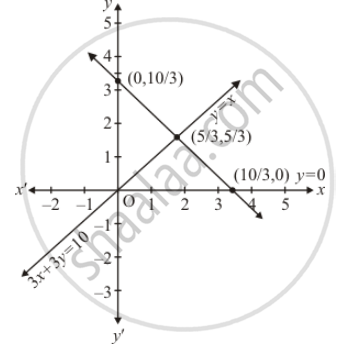Share

# Determine Graphically the Vertices of the Triangle, the Equations of Whose Sides Are Given Below : Y = X, Y = 0 and 3x + 3y = 10 - CBSE Class 10 - Mathematics

ConceptGraphical Method of Solution of a Pair of Linear Equations

#### Question

Determine graphically the vertices of the triangle, the equations of whose sides are given below :

y = xy = 0 and 3x + 3y = 10

#### Solution

Draw the 3 lines as given by equations

By taking x=1 = 1 cm on x−axis

And y =1=1cm on y−axis

y = 0

y = x

y/"10/3" + x/"10/3" = 1From graph point of intersection are (0,0) (10/3,0) (5/3,5/3)

Is there an error in this question or solution?

#### APPEARS IN

Solution Determine Graphically the Vertices of the Triangle, the Equations of Whose Sides Are Given Below : Y = X, Y = 0 and 3x + 3y = 10 Concept: Graphical Method of Solution of a Pair of Linear Equations.
S